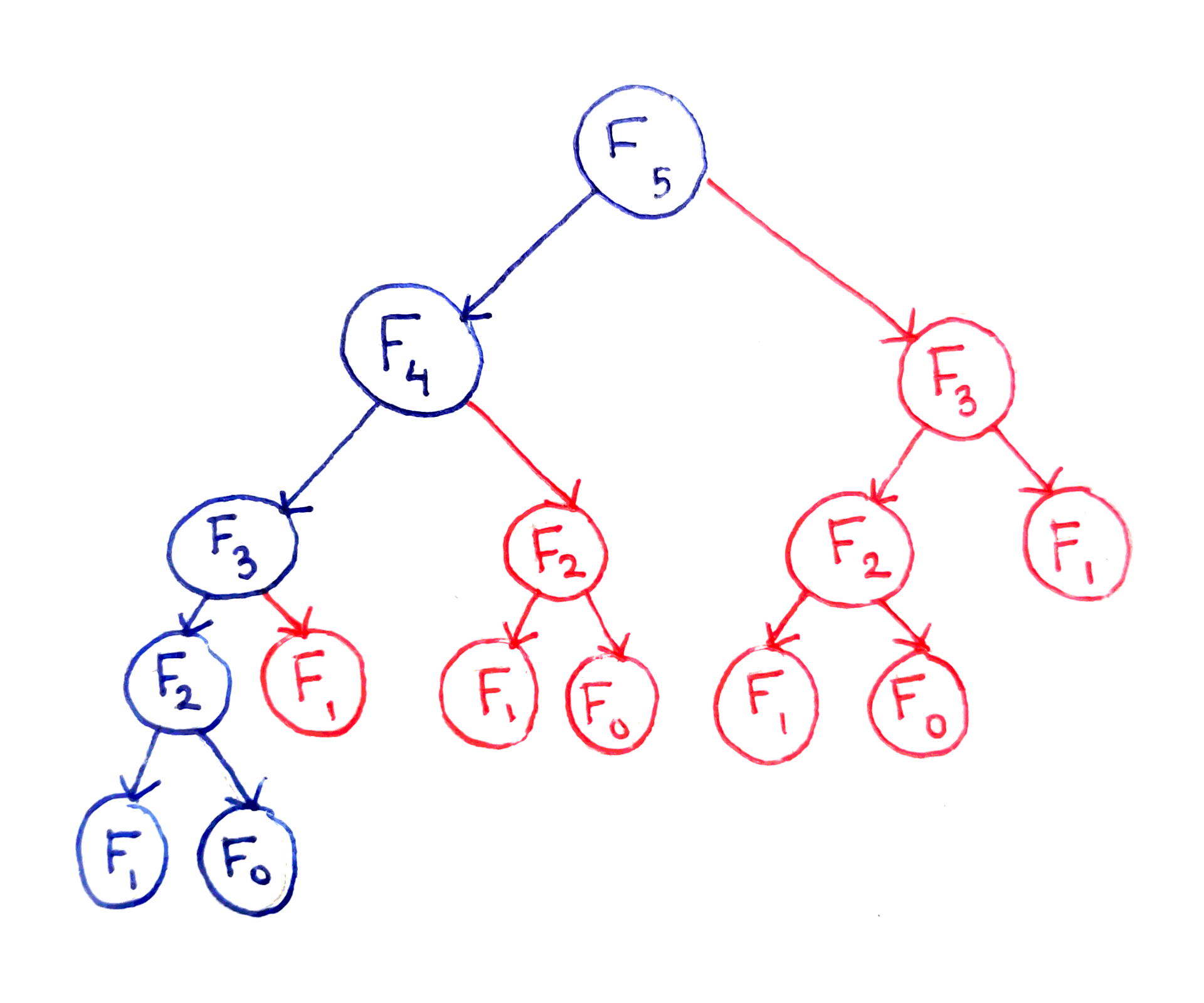### Algorithm

Everything that we do with a computer relies in some way on an algorithm. Any software (even the most advanced Artificial Intelligence) are only algorithms put together with some structured data (cf. below).
That's it: Algorithms + Data Structures = Software.In other words, it is a list of instructions, procedures, or formula that solves a problem… It can be a code to sort search results efficiently, a code to find the shortest route between A and B on a map, a formulal to encrypt and decrypt sensitive information, etc.

### Data Structure

Data structures are the way we can store and retrieve data, they represent the knowledge to be organized in memory. No matter what problem are we solving, in one way or another we have to deal with them !Dead code is any section of the codebase that is never used by the application.

### Infinite loop - Endless loop

An infinite loop is a sequence of instructions that will continue endlessly, unless an external intervention occurs. It may be intentional, but most of the time, our breaking condition doesn’t work. The result of such infinite loop often results in a application that freezes (and consume most of our CPU which may then lead to general lags) or even crash.

### Functional Programming

Functional programming or FP is a programming paradigm. This is a style of building software applications. Some of its principles are the way of building a program by composing pure functions, avoiding shared state, mutable data, and side-effects.

### Garbage collection

When a program is running, it uses the computer’s memory. Garbage collection does exactly what it says, it looks for memory that can’t be used by the program anymore and frees it, allowing this freed space to be used again.

### Memoization

Memoization is a technique used to store, the result of a computationally expensive function by storing it in a cache. If the function is called later using the same arguments, the result is loaded from the cache rather than being recomputed, saving CPU resources.

In brief: Memoization is a way to remember what we already found.
It is used to lower a function’s time cost in exchange for space cost.Where naive recursion falls short with Fibonacci: the tree above shows for each index (root) the nodes to be computed (all of its children). The red nodes are showing useless computation which may instead use the results already computed by the blue ones.

As a reminder the Fibonacci numbers are defined as follow:
F(0) = 0
F(1) = 1
F(n) = F(n−1) + F(n−2)

### Parse

The action of transforming data described textually into data stored within a data structure. When we fetch data from a web API, it is usually a JSON (just a string). We need to parse it, to transform it into an object, to manipulate data efficiently:

  // JSON data (stored as a string)
{
"name": "Maze Solver - Pathfinding",
"list": {
"a_star": "A* (A-Star)",
"dfs": "Depth First Search",
"dijkstra": "Dijkstra"
}
}

  // JS manipulate data through an Object built from the string parse.
const parsedData = JSON.parse(data);

// parsedData is now an object and can be accessed as follows:
parsedData.name;              // "Maze Solver - Pathfinding"
parseData.list.forEach(...);  // Iterate over the list


### Spaghetti code

Spaghetti code is a slang used to describe a program’s source code that is difficult to read or follow by a human because of how the original programmer wrote the code. It can be caused by several factors, such as volatile project requirements, lack of programming style rules, and insufficient ability or experience of the programmer.### Ternary Operator

This is a way of shortening a simple if-else block:

  const isTrue = (truth) ? "yes" : "no";


### Unary operator

A unary operator is an operator that takes only one value for its operation.

  auto x = 1; // x equal 1
++x;        // x is now equal to 2